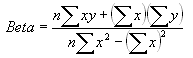Beta Calculation

Beta is the sensitivity of an investment's returns as compared to a benchmark.  This benchmark is commonly the S&P 500, but Fund Manager allows you to assign any investment as the benchmark.  See the Benchmark Dialog.  A benchmark must be assigned before beta calculations can be made.

A beta value less than 1 indicates the investment is less volatile than the benchmark.  A beta value equal to 1 means the investment's volatility is the same as the benchmark, and a beta greater than 1 means the investment is more volatile.

#### Calculation Details:

When calculating the beta of an investment the simple monthly returns over the specified comparison period are calculated.  The simple monthly return is:

Return = (End_price + Dist_per_share - Start_price) / Start_price

The returns for both the investment and benchmark are calculated.  If the comparison period is 5 years, there are 60 monthly returns for each the investment and the benchmark.  To calculate the beta, these 60 data points are plotted with the benchmark returns along the X axis, and the investment returns along the Y axis. The slope of a best-fit line through these data points is the Beta.where 'n' is the number of data points (60 in this example), and 'x' are the benchmark returns, and 'y' are the investment returns.  When calculating returns your current interpolation range preferences are used.  If sufficient pricing data is not available the Beta will not be reported.

The beta for a portfolio, asset type, goal, sector, or investment type is a weighted average of the beta's from the investments in that group.  The weighting is based on the ending value.  All investments with a non-zero ending value in that group must have a valid beta (sufficient pricing data) for that group beta to be reported.

Fund Manager can output the data points used in this calculation to a log file.  By default the logging feature is turned off.  To turn it on, create a new registry string value at:

HKEY_CURRENT_USER\Software\Beiley Software\Fund Manager\CurrentVersion\techanal

named "benchmarklog" and set it to a value of "1".  The log file is named "stats_log.txt", and will be located in your user's AppData\Roaming\Fund Manager folder (usually a hidden folder).  This log file is deleted upon exiting Fund Manager.